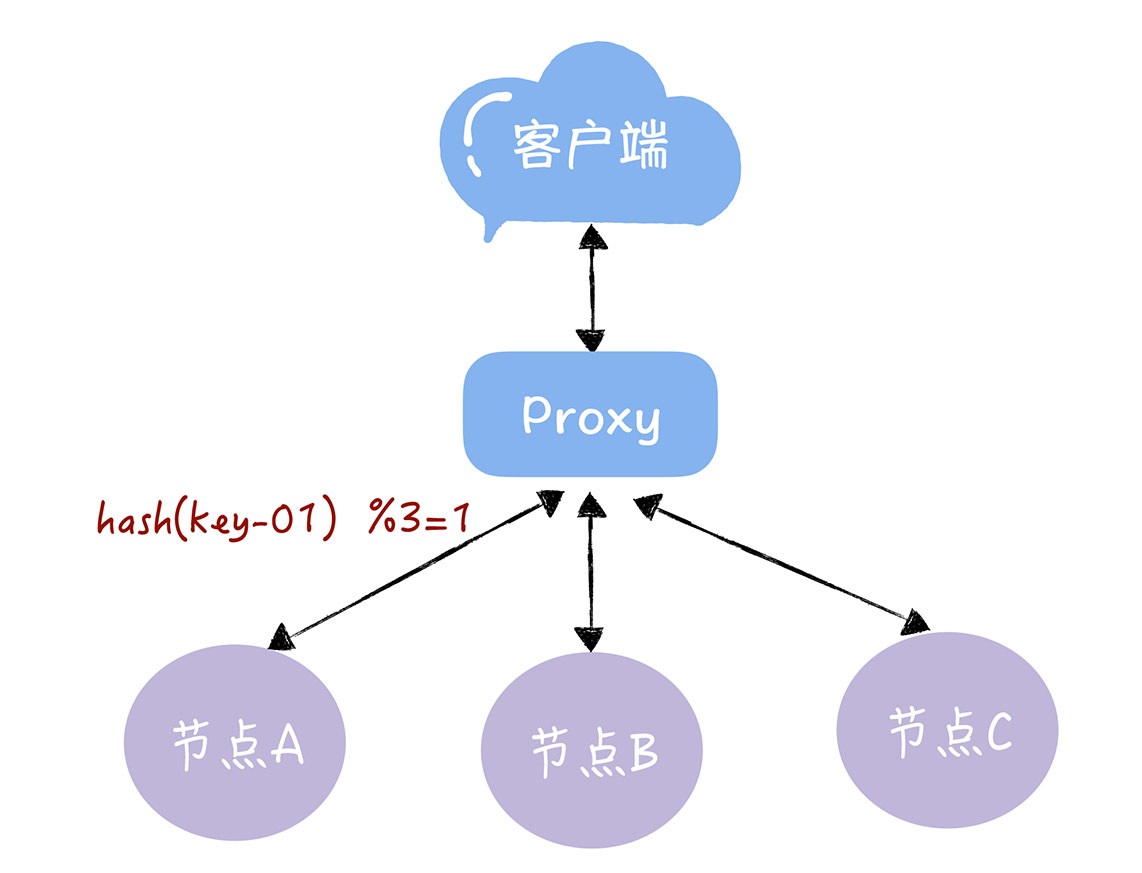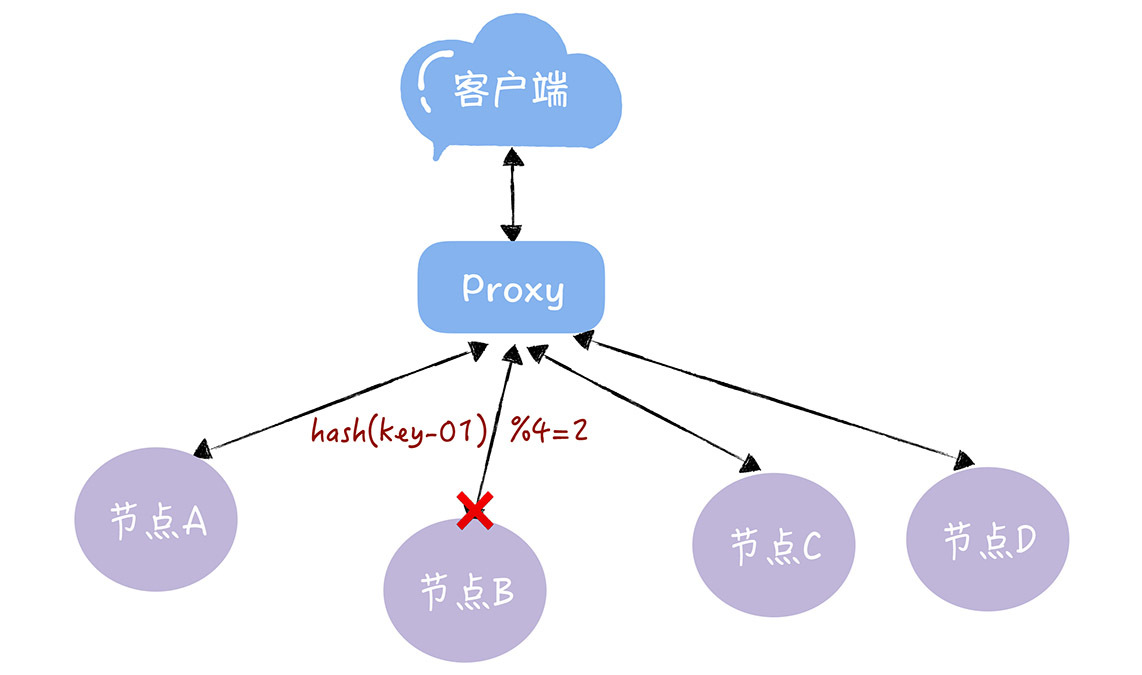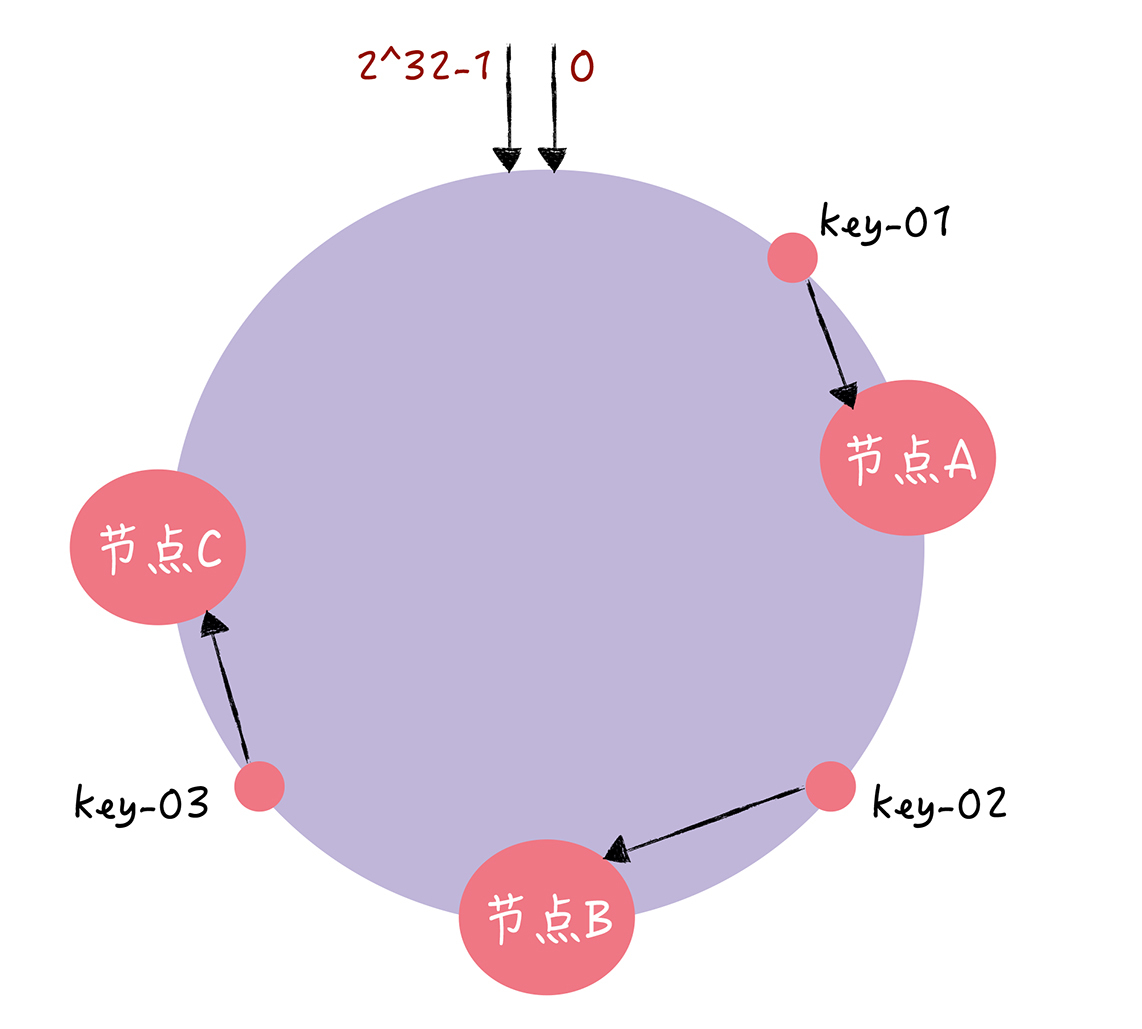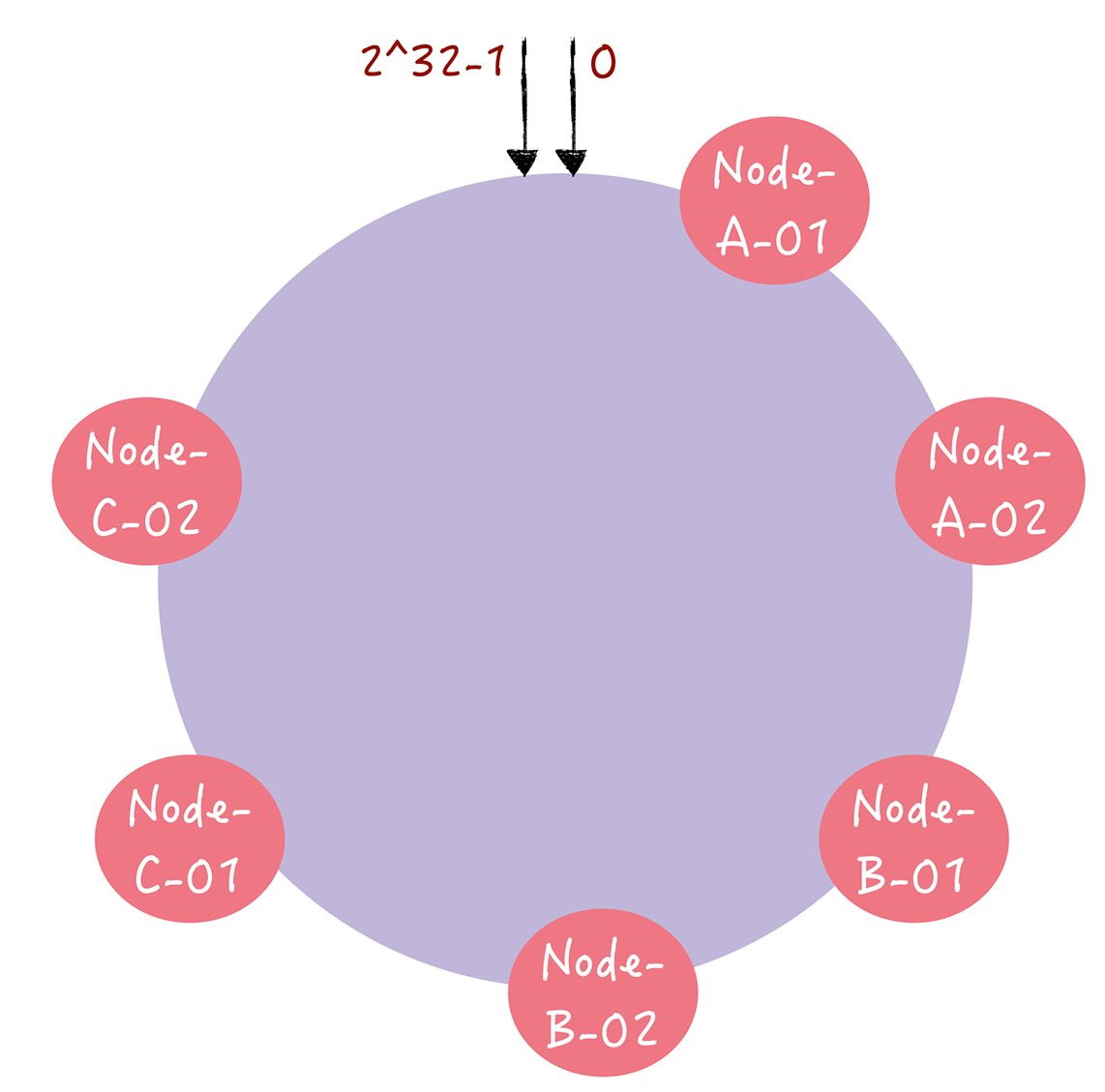# 【架构师训练营】week 5 homeworkeazonshaw# 前言

## 哈希寻址## 实现

/** * 哈希函数（取模） * @param key 键值 * @param nodes 节点数 * @return 哈希值 */private static int hash(int key,int nodes){    return key % nodes;}//mainint keys = 10000000;int nodes = 3;int newNodes = 4;Integer migrate = 0;for(int i = 0;i < keys;i++){    if(hash(i,nodes) != hash(i,newNodes)){        migrate++;    }}float migrateRatio = migrate.floatValue()/keys;System.out.println(migrateRatio*100+"%");

# 引入

1. 首先，将 key 作为参数执行 c-hash() 计算哈希值，并确定此 key 在环上的位置；

2. 然后，从这个位置沿着哈希环顺时针“行走”，遇到的第一节点就是 key 对应的节点。﻿

# 优化# 实现

## 构建哈希环

private final SortedMap<Long, T> circle = new TreeMap<>();

## 虚拟节点

﻿

//每个节点的虚拟节点的个数private final int numberOfReplicas;//初始化public ConsistentHash(int numberOfReplicas,Collection<T> nodes) {    this.numberOfReplicas = numberOfReplicas;    //初始化节点    for(T node:nodes)        add(node);}//添加节点public void add(T node){    for (int i = 0; i < numberOfReplicas; i++){        String key = node.toString()+i;        long hash = hash(key);        circle.put(hash,node);    }}

﻿

## 哈希函数

//哈希函数private static int hash(Object key){    return MurmurHash.hash32(key.toString());}

﻿

## 测试用例

//节点列表Integer numOfNodes = 10;String node = "10.0.0.";List<String> nodeList = new ArrayList<>();for(int i = 0;i < numOfNodes;i++){    nodeList.add(node+i);}System.out.println("节点数："+numOfNodes);//虚拟节点int replicas = 150;System.out.println("虚拟节点数："+replicas);//一致性哈希初始化ConsistentHash<String> consistentHash =        new ConsistentHash<>(replicas,nodeList);//KV数据int sampleCnt = 1000000;Map<String, Integer> counter = new ConcurrentHashMap<>();for (int i = 0; i < sampleCnt; i++) {    String latest = consistentHash.get(i);    counter.put(latest, counter.containsKey(latest) ? counter.get(latest) + 1 : 1);}//计算标准差double avg;double sum = 0;double standarddevSum = 0;double standarddev = 0;for(Map.Entry<String,Integer> entry:counter.entrySet()){    System.out.println("节点:"+entry.getKey()+"-数目："+entry.getValue());    sum += entry.getValue();}avg = sum/numOfNodes;for(Map.Entry<String,Integer> entry:counter.entrySet()){    standarddevSum += Math.pow(entry.getValue()-avg,2);}standarddev = Math.sqrt(standarddevSum/numOfNodes);System.out.println("标准差为："+standarddev);

﻿

## 输出结果

﻿

节点数：10虚拟节点数：150节点:10.0.0.8-数目：121826节点:10.0.0.7-数目：93329节点:10.0.0.9-数目：102347节点:10.0.0.4-数目：90985节点:10.0.0.3-数目：115595节点:10.0.0.6-数目：96799节点:10.0.0.5-数目：93851节点:10.0.0.0-数目：99645节点:10.0.0.2-数目：92648节点:10.0.0.1-数目：92975标准差为：10012.214200665107

﻿## 评论